SVG Tiny 1.2 – 20081222

# 9 Basic Shapes

## 9.1 Introduction

SVG contains the following set of basic shape elements:

• rectangles (including optional rounded corners), created with the 'rect' element,
• circles, created with the 'circle' element,
• ellipses, created with the 'ellipse' element,
• straight lines, created with the 'line' element,
• polylines, created with the 'polyline' element, and
• polygons, created with the 'polygon' element.

Mathematically, these shape elements are equivalent to a 'path' element that would construct the same shape. The basic shapes may be stroked, and filled. All of the properties available for 'path' elements also apply to the basic shapes.

## 9.2 The 'rect' element

The 'rect' element defines a rectangle which is axis-aligned with the current user coordinate system. Rounded rectangles can be achieved by setting appropriate values for attributes 'rx' and 'ry'.

Schema: rect
```    <define name='rect'>
<element name='rect'>
<ref name='rect.AT'/>
<zeroOrMore><ref name='shapeCommon.CM'/></zeroOrMore>
</element>
</define>

<define name='rect.AT' combine='interleave'>
<ref name='svg.ShapeCommon.attr'/>
<ref name='svg.XYWH.attr'/>
<ref name='svg.RxRyCommon.attr'/>
</define>
```

Attribute definitions:

x = "<coordinate>"

The x-axis coordinate of the side of the rectangle which has the smaller x-axis coordinate value in the current user coordinate system. The lacuna value is '0'.

Animatable: yes.

y = "<coordinate>"

The y-axis coordinate of the side of the rectangle which has the smaller y-axis coordinate value in the current user coordinate system. The lacuna value is '0'.

Animatable: yes.

width = "<length>"

The width of the rectangle. A negative value is unsupported. A value of zero disables rendering of the element. The lacuna value is '0'.

Animatable: yes.

height = "<length>"

The height of the rectangle. A negative value is unsupported. A value of zero disables rendering of the element. The lacuna value is '0'.

Animatable: yes.

rx = "<length>"

For rounded rectangles, the x-axis radius of the ellipse used to round off the corners of the rectangle. A negative value is unsupported. See the notes below about what happens if the attribute is not specified.

Animatable: yes.

ry = "<length>"

For rounded rectangles, the y-axis radius of the ellipse used to round off the corners of the rectangle. A negative value is unsupported. See the notes below about what happens if the attribute is not specified.

Animatable: yes.

focusable = "true" | "false" | "auto"

See attribute definition for description.

Animatable: yes.

See definition.

If a properly specified value is provided for 'rx' but not for 'ry', then the user agent must process the 'rect' element with the effective value for 'ry' as equal to 'rx'. If a properly specified value is provided for 'ry' but not for 'rx', then the user agent must process the 'rect' element with the effective value for 'rx' as equal to 'ry'. If neither 'rx' nor 'ry' has a properly specified value, then the user agent must process the 'rect' element as if no rounding had been specified, resulting in square corners. If 'rx' is greater than half of the width of the rectangle, then the user agent must process the 'rect' element with the effective value for 'rx' as half of the width of the rectangle. If 'ry' is greater than half of the height of the rectangle, then the user agent must process the 'rect' element with the effective value for 'ry' as half of the height of the rectangle.

A 'rect' element, taking its rounded corners into account, must be rendered in a way that produces the same result as if the following outline were specified instead (note: all coordinate and length values are first converted into user space coordinates according to Units):

1. Perform an absolute moveto operation to location (x+rx,y), where x and y are the values of the 'rect' element's 'x' and 'y' attribute converted to user space, and rx and ry are the effective values of the 'rx' and 'ry' attributes converted to user space.
2. Perform an absolute horizontal lineto operation to location (x+width-rx,y), where width is the 'rect' element's 'width' attribute converted to user space.
3. Perform an absolute elliptical arc operation to coordinate (x+width,y+ry), where the effective values for the 'rx' and 'ry' attributes on the 'rect' element converted to user space are used as the semimajor and semiminor axis, respectively, zero x-axis-rotation, a clockwise sweep direction and choosing the smaller arc sweep.
4. Perform an absolute vertical lineto operation to location (x+width,y+height-ry), where height is the 'rect' element's 'height' attribute converted to user space.
5. Perform an absolute elliptical arc operation to coordinate (x+width-rx,y+height).
6. Perform an absolute horizontal lineto operation to location (x+rx,y+height).
7. Perform an absolute elliptical arc operation to coordinate (x,y+height-ry).
8. Perform an absolute vertical lineto operation to location (x,y+ry).
9. Perform an absolute elliptical arc operation to coordinate (x+rx,y).
10. Perform a closepath (z) to the coordinate specified in the original moveto operation.

In case the 'rx' and 'ry' attributes are not specified or set to a value of zero, the elliptical arc commands should be omitted.

Example 09_01 shows a rectangle with sharp corners. The 'rect' element is filled with yellow and stroked with navy.

Example: 09_01.svg
```<?xml version="1.0"?>
<svg width="12cm" height="4cm" viewBox="0 0 1200 400"
xmlns="http://www.w3.org/2000/svg" version="1.2" baseProfile="tiny">
<desc>Example rect01 - rectangle with sharp corners</desc>
<!-- Show outline of canvas using 'rect' element -->
<rect x="1" y="1" width="1198" height="398"
fill="none" stroke="blue" stroke-width="2"/>
<rect x="400" y="100" width="400" height="200"
fill="yellow" stroke="navy" stroke-width="10"  />
</svg>
```Example 09_02 shows two rounded rectangles. The 'rx' specifies how to round the corners of the rectangles. Note that since no value has been specified for the 'ry' attribute, it will be assigned the same value as the 'rx' attribute.

Example: 09_02.svg
```<?xml version="1.0"?>
<svg width="12cm" height="4cm" viewBox="0 0 1200 400"
xmlns="http://www.w3.org/2000/svg" version="1.2" baseProfile="tiny">
<desc>Example rect02 - rounded rectangles</desc>
<!-- Show outline of canvas using 'rect' element -->
<rect x="1" y="1" width="1198" height="398"
fill="none" stroke="blue" stroke-width="2"/>
<rect x="100" y="100" width="400" height="200" rx="50"
fill="green" />
<g transform="translate(700 210) rotate(-30)">
<rect x="0" y="0" width="400" height="200" rx="50"
fill="none" stroke="purple" stroke-width="30" />
</g>
</svg>
```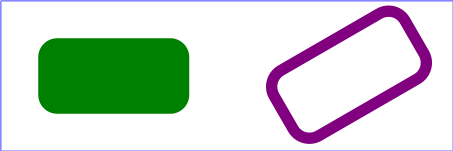## 9.3 The 'circle' element

The 'circle' element defines a circle based on a center point and a radius.

Within the current user coordinate system, stroking operations on a circle begin at the point (cx+r,cy) and then proceed through the points (cx,cy+r), (cx-r,cy), (cx,cy-r) and finally back to (cx+r,cy). For stroking operations, there is only one line segment which has its beginning joined to its end.

Schema: circle
```    <define name='circle'>
<element name='circle'>
<ref name='circle.AT'/>
<zeroOrMore><ref name='shapeCommon.CM'/></zeroOrMore>
</element>
</define>

<define name='circle.AT' combine='interleave'>
<ref name='svg.ShapeCommon.attr'/>
<ref name='svg.CxCy.attr'/>
<ref name='svg.R.attr'/>
</define>
```

Attribute definitions:

cx = "<coordinate>"

The x-axis coordinate of the center of the circle. The lacuna value is '0'.

Animatable: yes.

cy = "<coordinate>"

The y-axis coordinate of the center of the circle. The lacuna value is '0'.

Animatable: yes.

r = "<length>"

The radius of the circle. A negative value is unsupported. A value of zero disables rendering of the element. The lacuna value is '0'.

Animatable: yes.

focusable = "true" | "false" | "auto"

See attribute definition for description.

Animatable: yes.

See definition.

Example circle01 consists of a 'circle' element that is filled with red and stroked with blue.

Example: 09_03.svg
```<?xml version="1.0"?>
<svg width="12cm" height="4cm" viewBox="0 0 1200 400"
xmlns="http://www.w3.org/2000/svg" version="1.2" baseProfile="tiny">
<desc>Example circle01 - circle filled with red and stroked with blue</desc>
<!-- Show outline of canvas using 'rect' element -->
<rect x="1" y="1" width="1198" height="398"
fill="none" stroke="blue" stroke-width="2"/>
<circle cx="600" cy="200" r="100"
fill="red" stroke="blue" stroke-width="10"  />
</svg>
```## 9.4 The 'ellipse' element

The 'ellipse' element defines an ellipse which is axis-aligned with the current user coordinate system based on a center point and two radii.

Within the current user coordinate system, stroking operations on a ellipse begin at the point (cx+rx,cy) and then proceed through the points (cx,cy+ry), (cx-rx,cy), (cx,cy-ry) and finally back to (cx+rx,cy). For stroking operations, there is only one line segment which has its beginning joined to its end.

Schema: ellipse
```    <define name='ellipse'>
<element name='ellipse'>
<ref name='ellipse.AT'/>
<zeroOrMore><ref name='shapeCommon.CM'/></zeroOrMore>
</element>
</define>

<define name='ellipse.AT' combine='interleave'>
<ref name='svg.ShapeCommon.attr'/>
<ref name='svg.RxRyCommon.attr'/>
<ref name='svg.CxCy.attr'/>
</define>
```

Attribute definitions:

cx = "<coordinate>"

The x-axis coordinate of the center of the ellipse. The lacuna value is '0'.

Animatable: yes.

cy = "<coordinate>"

The y-axis coordinate of the center of the ellipse. The lacuna value is '0'.

Animatable: yes.

rx = "<length>"

The x-axis radius of the ellipse. A negative value is unsupported. A value of zero disables rendering of the element. The lacuna value is '0'.

Animatable: yes.

ry = "<length>"

The y-axis radius of the ellipse. A negative value is unsupported. A value of zero disables rendering of the element. The lacuna value is '0'.

Animatable: yes.

focusable = "true" | "false" | "auto"

See attribute definition for description.

Animatable: yes.

See definition.

Example 09_04 below specifies the coordinates of the two ellipses in the user coordinate system established by the 'viewBox' attribute on the 'svg' element and the 'transform' attribute on the 'g' and 'ellipse' elements. Both ellipses use the lacuna value of zero for the 'cx' and 'cy' attributes (the center of the ellipse). The second ellipse is rotated.

Example: 09_04.svg
```<?xml version="1.0"?>
<svg width="12cm" height="4cm" viewBox="0 0 1200 400"
xmlns="http://www.w3.org/2000/svg" version="1.2" baseProfile="tiny">
<desc>Example ellipse01 - examples of ellipses</desc>
<!-- Show outline of canvas using 'rect' element -->
<rect x="1" y="1" width="1198" height="398"
fill="none" stroke="blue" stroke-width="2" />
<g transform="translate(300 200)">
<ellipse rx="250" ry="100"
fill="red"  />
</g>
<ellipse transform="translate(900 200) rotate(-30)"
rx="250" ry="100"
fill="none" stroke="blue" stroke-width="20"  />
</svg>
```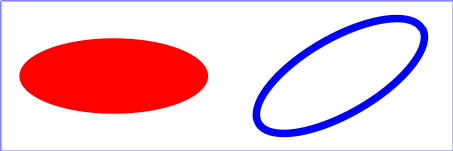## 9.5 The 'line' element

The 'line' element defines a line segment that starts at one point and ends at another.

Schema: line
```    <define name='line'>
<element name='line'>
<ref name='line.AT'/>
<zeroOrMore><ref name='shapeCommon.CM'/></zeroOrMore>
</element>
</define>

<define name='line.AT' combine='interleave'>
<ref name='svg.ShapeCommon.attr'/>
<ref name='svg.X12Y12.attr'/>
</define>
```

Attribute definitions:

x1 = "<coordinate>"

The x-axis coordinate of the start of the line. The lacuna value is '0'.

Animatable: yes.

y1 = "<coordinate>"

The y-axis coordinate of the start of the line. The lacuna value is '0'.

Animatable: yes.

x2 = "<coordinate>"

The x-axis coordinate of the end of the line. The lacuna value is '0'.

Animatable: yes.

y2 = "<coordinate>"

The y-axis coordinate of the end of the line. The lacuna value is '0'.

Animatable: yes.

focusable = "true" | "false" | "auto"

See attribute definition for description.

Animatable: yes.

See definition.

A 'line' element must be rendered in a way that produces the same result as if the following path were specified instead (note: all coordinate and length values are first converted into user space coordinates according to Units):

1. Perform an absolute moveto operation to absolute location (x1,y1), where x1 and y1 are the values of the 'line' element's 'x1' and 'y1' attributes converted to user space, respectively.
2. Perform an absolute lineto operation to absolute location (x2,y2), where x2 and y2 are the values of the 'line' element's 'x2' and 'y2' attributes converted to user space, respectively.

Because 'line' elements are single lines and thus are geometrically one-dimensional, they have no interior; thus, 'line' elements are never filled (see the 'fill' property).

Example 09_05 below specifies the coordinates of the five lines in the user coordinate system established by the 'viewBox' attribute on the 'svg' element. The lines have different thicknesses.

Example: 09_05.svg
```<?xml version="1.0"?>
<svg width="12cm" height="4cm" viewBox="0 0 1200 400"
xmlns="http://www.w3.org/2000/svg" version="1.2" baseProfile="tiny">
<desc>Example line01 - lines expressed in user coordinates</desc>
<!-- Show outline of canvas using 'rect' element -->
<rect x="1" y="1" width="1198" height="398"
fill="none" stroke="blue" stroke-width="2" />
<g stroke="green" >
<line x1="100" y1="300" x2="300" y2="100"
stroke-width="5"  />
<line x1="300" y1="300" x2="500" y2="100"
stroke-width="10"  />
<line x1="500" y1="300" x2="700" y2="100"
stroke-width="15"  />
<line x1="700" y1="300" x2="900" y2="100"
stroke-width="20"  />
<line x1="900" y1="300" x2="1100" y2="100"
stroke-width="25"  />
</g>
</svg>
```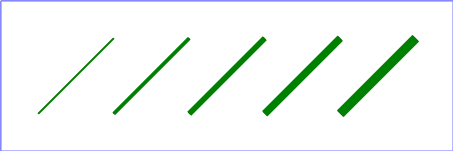## 9.6 The 'polyline' element

The 'polyline' element defines a set of connected straight line segments. Typically, 'polyline' elements define open shapes.

Schema: polyline
```    <define name='polyline'>
<element name='polyline'>
<ref name='polyCommon.AT'/>
<zeroOrMore><ref name='shapeCommon.CM'/></zeroOrMore>
</element>
</define>

<define name='polyCommon.AT' combine='interleave'>
<ref name='svg.ShapeCommon.attr'/>
<optional>
<attribute name='points' svg:animatable='true' svg:inheritable='false'>
<ref name='Points.datatype'/>
</attribute>
</optional>
</define>
```

Attribute definitions:

points = "<points-data>"

The points that make up the polyline. All coordinate values are in the user coordinate system.

An empty attribute value (points="") disables rendering of the element. The lacuna value is the empty string.

Animatable: yes.

focusable = "true" | "false" | "auto"

See attribute definition for description.

Animatable: yes.

See definition.

If an odd number of coordinates is provided, then the element is treated as if the attribute had not been specified.

A 'polyline' element must be rendered in a way that produces the same result as if the following path were specified instead:

1. Perform an absolute moveto operation to the first coordinate pair in the list of points.
2. For each subsequent coordinate pair, perform an absolute lineto operation to that coordinate pair.

Example 09_06 below specifies a polyline in the user coordinate system established by the 'viewBox' attribute on the 'svg' element.

Example: 09_06.svg
```<?xml version="1.0"?>
<svg width="12cm" height="4cm" viewBox="0 0 1200 400"
xmlns="http://www.w3.org/2000/svg" version="1.2" baseProfile="tiny">
<desc>Example polyline01 - increasingly larger bars</desc>
<!-- Show outline of canvas using 'rect' element -->
<rect x="1" y="1" width="1198" height="398"
fill="none" stroke="blue" stroke-width="2" />
<polyline fill="none" stroke="blue" stroke-width="10"
points="50,375
150,375 150,325 250,325 250,375
350,375 350,250 450,250 450,375
550,375 550,175 650,175 650,375
750,375 750,100 850,100 850,375
950,375 950,25 1050,25 1050,375
1150,375" />
</svg>```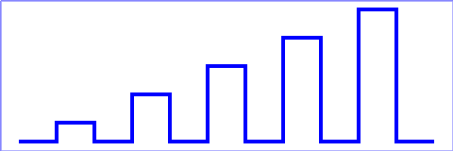## 9.7 The 'polygon' element

The 'polygon' element defines a closed shape consisting of a set of connected straight line segments.

Schema: polygon
```    <define name='polygon'>
<element name='polygon'>
<ref name='polyCommon.AT'/>
<zeroOrMore><ref name='shapeCommon.CM'/></zeroOrMore>
</element>
</define>
```

Attribute definitions:

points = "<points-data>"

The points that make up the polygon. All coordinate values are in the user coordinate system.

An empty attribute value (points="") disables rendering of the element. The lacuna value is the empty string.

Animatable: yes.

focusable = "true" | "false" | "auto"

See attribute definition for description.

Animatable: yes.

See definition.

If an odd number of coordinates is provided in the 'points' attribute, then it is treated as an unsupported value.

A 'polygon' element must be rendered in a way that produces the same result as if the following path were specified instead:

1. Perform an absolute moveto operation to the first coordinate pair in the list of points.
2. For each subsequent coordinate pair, perform an absolute lineto operation to that coordinate pair.
3. Perform a closepath command.

Example 09_07 below specifies two polygons (a star and a hexagon) in the user coordinate system established by the 'viewBox' attribute on the 'svg' element.

Example: 09_07.svg
```<?xml version="1.0"?>
<svg width="12cm" height="4cm" viewBox="0 0 1200 400"
xmlns="http://www.w3.org/2000/svg" version="1.2" baseProfile="tiny">
<desc>Example polygon01 - star and hexagon</desc>
<!-- Show outline of canvas using 'rect' element -->
<rect x="1" y="1" width="1198" height="398"
fill="none" stroke="blue" stroke-width="2" />
<polygon fill="red" stroke="blue" stroke-width="10"
points="350,75  379,161 469,161 397,215
423,301 350,250 277,301 303,215
231,161 321,161" />
<polygon fill="lime" stroke="blue" stroke-width="10"
points="850,75  958,137.5 958,262.5
850,325 742,262.6 742,137.5" />
</svg>
```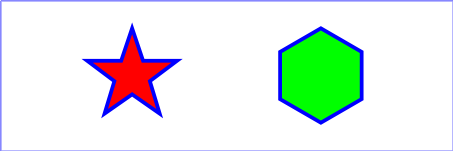### 9.7.1 The grammar for points specifications in 'polyline' and 'polygon' elements

The following is an EBNF grammar for <points-data> values on 'polyline' and 'polygon' elements [EBNF]:

```points-data:
wsp* coordinate-pairs? wsp*
coordinate-pairs:
coordinate-pair
| coordinate-pair comma-wsp coordinate-pairs
coordinate-pair:
coordinate comma-wsp coordinate
coordinate:
number
number:
sign? integer-constant
| sign? floating-point-constant
comma-wsp:
(wsp+ comma? wsp*) | (comma wsp*)
comma:
","
integer-constant:
digit-sequence
floating-point-constant:
fractional-constant exponent?
| digit-sequence exponent
fractional-constant:
digit-sequence? "." digit-sequence
| digit-sequence "."
exponent:
( "e" | "E" ) sign? digit-sequence
sign:
"+" | "-"
digit-sequence:
digit
| digit digit-sequence
digit:
"0" | "1" | "2" | "3" | "4" | "5" | "6" | "7" | "8" | "9"
wsp:
(#x20 | #x9 | #xD | #xA)+
```